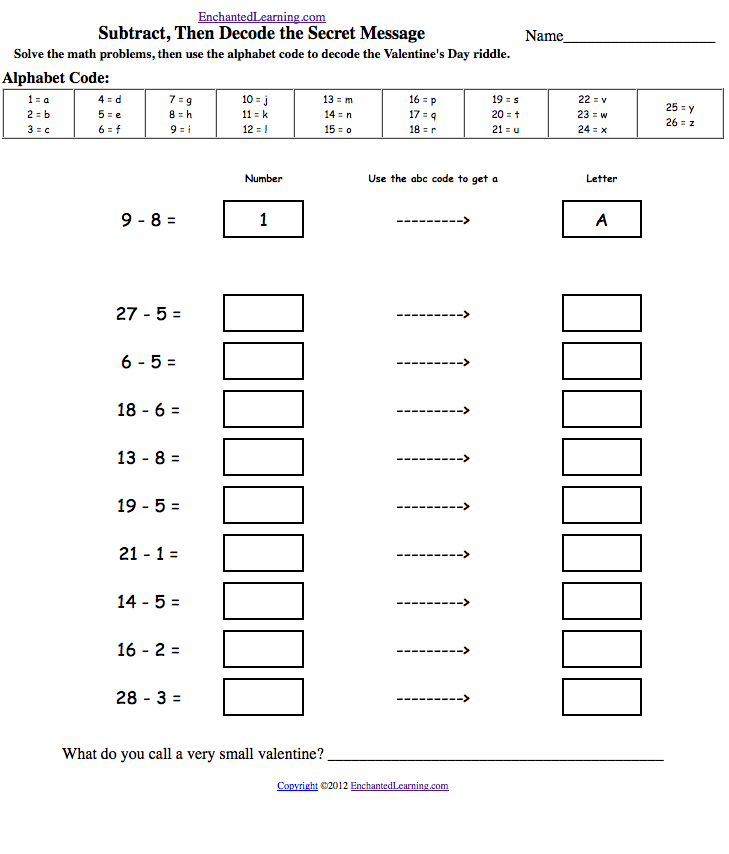EnchantedLearning.com is a user-supported site.
As a bonus, site members have access to a banner-ad-free version of the site, with print-friendly pages.

 You might also like: Banana Shoe Joke: Add and Subtract, Then Decode the Riddle: Arithmetic Worksheet Clown Joke: Add and Subtract, Then Decode the Riddle: Arithmetic Worksheet Man-in-the-Moon Joke: Add and Subtract, Then Decode the Riddle: Arithmetic Worksheet Owl Jokes: Add and Subtract, Then Decode the Riddle: Arithmetic Worksheet Solve, Then Decode: Subtraction Worksheets Today's featured page: Francisco Pizarro - Biography
 More Math EnchantedLearning.comSolve, Then Decode:Arithmetic Worksheets In these theme-based printable puzzles, the student first solves simple arithmetic problems, then uses the alphabet code to decode a riddle, quote, or secret message. Alphabet PagesBanana Shoe Riddle: Add and Subtract, then Decode the RiddleSolve the addition and subtraction problems, then use the alphabet code to answer the silly riddle, "What do you call a shoe made from banana peels? (Answer - A slipper). Or go to the answer page.Clown Riddle: Add and Subtract, then Decode the RiddleSolve the addition and subtraction problems, then use the alphabet code to answer the clown riddle, "Why did the clown go to the doctor?" (Answer - He was feeling funny). Or go to the answer page.Leopard Riddle: Add and Subtract, then Decode the RiddleSolve the addition and subtraction problems, then use the alphabet code to answer the leopard riddle, "Which side of a leopard has the most spots?" (Answer - The outside). Or go to the answer page.Man-in-the-Moon Riddle: Add and Subtract, then Decode the RiddleSolve the addition and subtraction problems, then use the alphabet code to answer the man-in-the-moon riddle, "How does the man-in-the-moon cut his hair?" (Answer - Eclipse it). Or go to the answer page.Owl Riddles:Add and Subtract, then Decode the RiddlesSolve the addition and subtraction problems, then use the alphabet code to answer the owl riddles, "What do you call an owl with a deep voice?" (Answer - A growl), and "Someone said you sound like an owl." (Answer - Who?). Or go to the answer page.Penguin Money Riddle:Add and Subtract, then Decode the RiddleSolve the addition and subtraction problems, then use the alphabet code to answer the penguin riddles, "Where do penguins keep their money?" (Answer - In a snow bank). Or go to the answer page.Raining Cats and Dogs Riddle: Add and Subtract, then Decode the RiddleSolve the addition and subtraction problems, then use the alphabet code to answer the weather riddle, "What's worse than raining cats and dogs? (Answer - Hailing taxis). Or go to the answer page.Snow Boots Riddle: Add and Subtract, then Decode the RiddleSolve the addition and subtraction problems, then use the alphabet code to answer the snow boots riddle, "What's the biggest problem with snow boots? (Answer - They melt). Or go to the answer page.Twenty-eight Days Riddle: Add and Subtract, then Decode the RiddleSolve the addition and subtraction problems, then use the alphabet code to answer the calendar riddle, "How many months have 28 days? (Answer - All of them). Or go to the answer page.US Constitution Riddle: Add and Subtract, then Decode the RiddleSolve the addition and subtraction problems, then use the alphabet code to answer the US Constitution riddle, "Where was the US Constitution signed? (Answer - At the bottom). Or go to the answer page.

Enchanted Learning®
Over 35,000 Web Pages
Sample Pages for Prospective Subscribers, or click below

 Overview of Site What's New Enchanted Learning Home Monthly Activity Calendar Books to Print Site Index K-3 Crafts K-3 Themes Little ExplorersPicture dictionary PreK/K Activities Rebus Rhymes Stories Writing Cloze Activities Essay Topics Newspaper Writing Activities Parts of Speech Fiction The Test of Time iPhone app TapQuiz Maps - free iPhone Geography Game Biology Animal Printouts Biology Label Printouts Biomes Birds Butterflies Dinosaurs Food Chain Human Anatomy Mammals Plants Rainforests Sharks Whales Physical Sciences: K-12 Astronomy The Earth Geology Hurricanes Landforms Oceans Tsunami Volcano Languages Dutch French German Italian Japanese (Romaji) Portuguese Spanish Swedish Geography/History Explorers Flags Geography Inventors US History Other Topics Art and Artists Calendars College Finder Crafts Graphic Organizers Label Me! Printouts Math Music Word Wheels

## Enchanted Learning Search

 Search the Enchanted Learning website for: